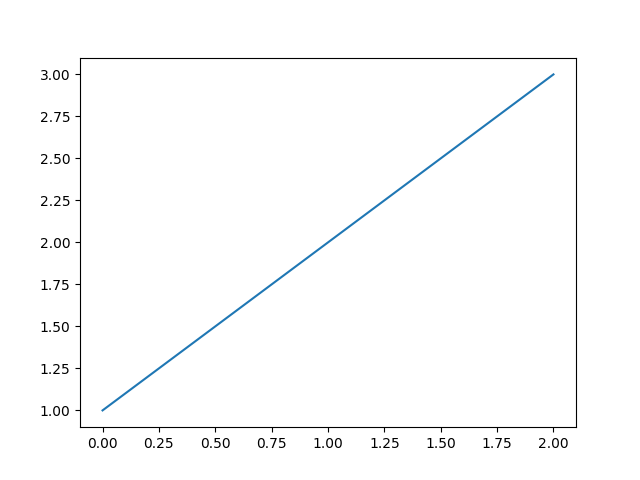# Agg Buffer¶

Use backend agg to access the figure canvas as an RGB string and then convert it to an array and pass it to Pillow for rendering.```import numpy as np

from matplotlib.backends.backend_agg import FigureCanvasAgg
import matplotlib.pyplot as plt

plt.plot([1, 2, 3])

canvas = plt.get_current_fig_manager().canvas

agg = canvas.switch_backends(FigureCanvasAgg)
agg.draw()
s, (width, height) = agg.print_to_buffer()

# Convert to a NumPy array.
X = np.fromstring(s, np.uint8).reshape((height, width, 4))

# Pass off to PIL.
from PIL import Image
im = Image.frombytes("RGBA", (width, height), s)

# Uncomment this line to display the image using ImageMagick's `display` tool.
# im.show()
```

Keywords: matplotlib code example, codex, python plot, pyplot Gallery generated by Sphinx-Gallery